# Chapter 3 Pair of Linear Equation in Two Variables NCERT Exemplar Solutions Exercise 3.3 Class 10 Maths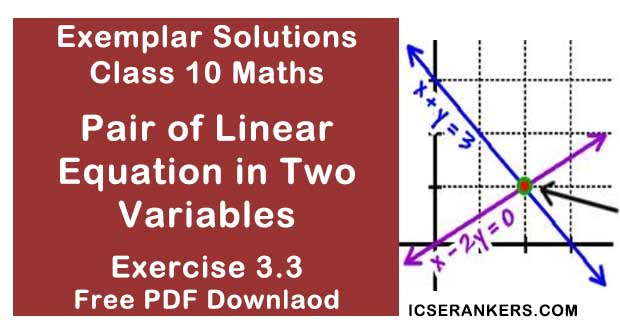Chapter Name NCERT Maths Exemplar Solutions for Chapter 3 Pair of Linear Equation in Two Variables Exercise 3.3 Book Name NCERT Exemplar for Class 10 Maths Other Exercises Exercise 3.1Exercise 3.2Exercise 3.4 Related Study NCERT Solutions for Class 10 Maths

### Exercise 3.3 Solutions

1. For which value(s) of λ, do the pair of linear equations λx + y = λ2 and x + λy = 1 have
(i) no solution?
(ii) infinitely many solutions?
(iii) a unique solution?
Solution
The given equations are;
λx + y = λ2 = 0
x + λy = 1
We have,
a1 = λ
b1 = 1,
c1 = - λ2 ,
a2 = 1,
b2 = λ
c2 = -1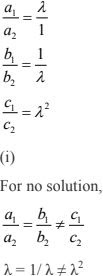So,  λ2 = 1and  λ2 ≠ λ
We take only λ = - 1,
Since the system of linear equations has infinitely many solution at λ = 1,

(ii) For infinitely many solutions,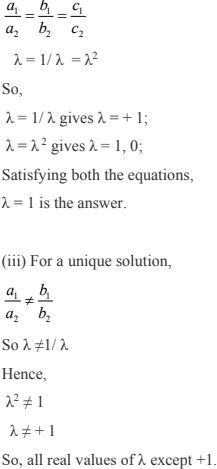2. For which value(s) of k will the pair of equations
kx + 3y = k – 3
12x + ky = k have no solution?
Solution
The given pair of linear equations is
kx + 3y = k – 3 ...(i)
12x + ky = k ...(ii)
We have,
a1 = k
b1 = 3,
c1 = - (k - 3),
a2 = 12,
b2 = k
c2 = -k
Then,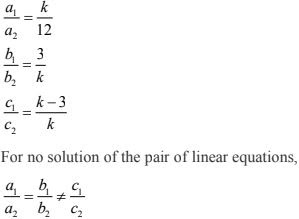k/12 = 3/k ≠ (k - 3)/k
Taking first two parts, we get
k/12 = 3/k ≠ (k-3)/k
Taking first two parts, we get
k/12 = 3/k
⇒ k2 = 36
⇒ k = + 6
Taking last two parts, we get
3/k = (k -3)k
⇒ 3k = k(k - 3)
⇒ k2 - 6k = 0
So, k ≠ 0, 6
Value of k for which the given pair of linear equations has no solution is k = -6.

3. For which values of a and b, will the following pair of linear equations have infinitely many solutions?
x + 2y = 1
(a – b)x + (a + b)y = a + b – 2
Solution
The given pair of linear equations are:
x + 2y = 1 ...(i)
(a-b)x + (a + b)y = a + b – 2 ...(ii)
We have,
a1 = 1,
b1 = 2,
c1 = -1,
a2 = (a - b),
b2 = (a + b),
c2 = -(a + b - 2)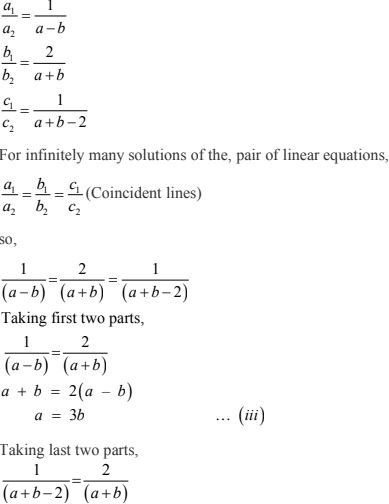2(a + b – 2) = (a + b)
a + b = 4 ...(iv)
Putting the value of a from Eq. (iii) in Eq. (iv), we get
3b + b = 4
⇒ 4b = 4
⇒ b = 1
Put the value of b in Eq. (iii), we get
a = 3
So, the values (a,b) = (3,1) satisfies all the parts.
Required values of a and b are 3 and 1 respectively for which the given pair of linear
equations has infinitely many solutions.

4. Find the value(s) of p in (i) to (iv) and p and q in (v) for the following pair of
equations:
(i) 3x – y – 5 = 0 and 6x – 2y – p = 0, if the lines represented by these equations are parallel.
Solution
Given pair of linear equations is
3x – y – 5 = 0 ...(i)
6x – 2y – p = 0 ...(ii)
We have,
a1 = 3,
b1 = -1,
c1 = -5,
a2 = 6,
b2 = -2,
c2 = -p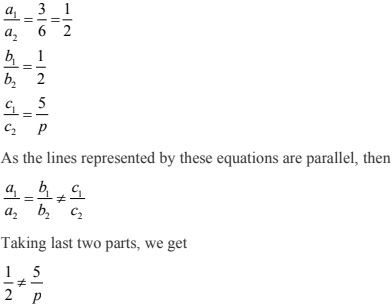So,
p ≠ 10
Hence, the given pair of linear equations are parallel for all real values of p except 10.

(ii) – x + py = 1 and px – y = 1, if the pair of equations has no solution.
Solution
Given pair of linear equations is
– x + py = 1 ...(i)
px – y – 1 = 0 ...(ii)
We have,
a1 = -1,
b1 = p,
c1 = -1,
a2 = p,
b2 = -1,
c2 = -1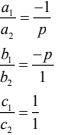Since, the lines equations has no solution i.e., both lines are parallel to each other.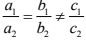-1/p = – p ≠ 1
Taking last two parts, we get
p ≠ -1
Taking first two parts, we get
p2 = 1
p = + 1
So, the given pair of linear equations has no solution for p = 1.

(iii) – 3x + 5y = 7 and 2px – 3y = 1, if the lines represented by these equations are intersecting at a unique point.
Solution
Given, pair of linear equations is
– 3x + 5y = 7
2px – 3y = 1
We have,
a1 = -3,
b1 = 5,
c1 = – 7;
And
a2 = 2p,
b2 = – 3,
c2 = – 1;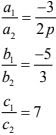Since, the lines are intersecting at a unique point i.e., it has a unique solution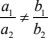-3/2p ≠ -5/3
p ≠ 9/10
Hence, the lines represented by these equations are intersecting at a unique point for all real
values of p except 9/10

(iv) 2x + 3y – 5 = 0 and px – 6y – 8 = 0, if the pair of equations has a unique solution.
Solution
Given, pair of linear equations is
2x + 3y – 5 = 0
px – 6y – 8 = 0
We have,
a1 = 2,
b1 = 3,
c1 = – 5;
And
a2 = p,
b2 = – 6,
c2 = – 8;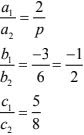Since, the pair of linear equations has a unique solution.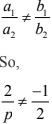p ≠ -4
so, the pair of linear equations has a unique solution for all values of p except - 4.

(v) 2x + 3y = 7 and 2px + py = 28 – qy, if the pair of equations have infinitely many solutions.
Solution
Given pair of linear equations is
2x + 3y = 7
2px + py = 28 – qy
2px + (p + q)y – 28 = 0
We have,
a1 = 2,
b1 = 3,
c1 = – 7;
And
a2 = 2p,
b2 = (p + q),
c2 = – 28;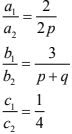Since, the pair of equations has infinitely many solutions i.e., both lines are coincident.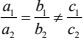Taking first and third parts, we get
p = 4
Again, taking last two parts, we get
3(p + q) = 1/4
⇒ p + q = 12
Since, p = 4
So, q = 8
We get that the values of p = 4 and q = 8 satisfies all three parts.
So, the pair of equations has infinitely many solutions for all values of p = 4 and q = 8.

5. Two straight paths are represented by the equations x – 3y = 2 and –2x + 6y = 5. Check whether the paths cross each other or not.
Solution
Linear equations are
x – 3y – 2 = 0 ...(i)
-2x + 6y – 5 = 0 ...(ii)
We have,
a1 =1,
b1 =-3,
c1 =- 2;
a2 = -2,
b2 = 6,
c2 = -5;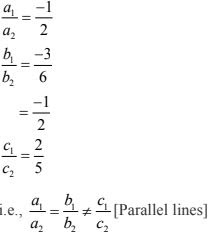The, two straight paths represented by the given equations never cross each other, because they are parallel to each other.

6. Write a pair of linear equations which has the unique solution x = – 1, y =3. How many such pairs can you write?
Solution
Condition for the pair of system to have unique solution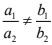Let the equations be,
a1x + b1y + c1 = 0
a2x + b2y + c2 = 0
Since, x = –1 and y = 3 is the unique solution of these two equations, then
It must satisfy the equations –
a1(-1) + b1(3) + c1  = 0
⇒ –a1 + 3b1 + c1 = 0 ...(i)
And
a2(- 1) + b2(3) + c2  = 0
⇒ –a2 + 3b2 + c2 = 0 ...(ii)
As for the different values of a1 , b1 , c1  and a2 , b2 , c2  satisfy the Eq. (i) and (ii).
Therefore, infinitely many pairs of linear equations are possible.

7. If 2x + y = 23 and 4x – y = 19, find the values of 5y – 2x and y/x – 2.
Solution
Given equations are
2x + y = 23 ...(i)
4x – y = 19 ...(ii)
On adding both equations, we get
6x = 42
So, x = 7
Putting the value of x in Eq. (i), we get
2(7) + y = 23
⇒ y = 23 – 14
⇒ y = 9
And,
5y – 2x = 5(9) – 2(7)
= 45 – 14
= 31
y/x – 2 = 9/7 -2
= -5/7
So, the values of (5y – 2x) and y/x – 2 are 31 and -5/7 respectively.

8. Find the values of x and y in the following rectangle [see Fig. 3.2].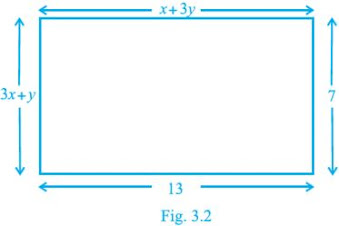Solution
Using property of rectangle,
We know that, Lengths are equal,
CD = AB
Therefore,
x + 3y = 13 ...(i)
3x + y = 7 ...(ii)
On multiplying Eq. (ii) by 3 and then subtracting Eq. (i),
We get,
8x = 8
⇒ x = 1
Putting x = 1 in Eq. (i),
y = 4
Therefore, the required values of x and y are 1 and 4, respectively.

9. Solve the following pairs of equations:
(i) x + y = 3.3
0.6(3x - 2y) = -1

(ii) x/3 + y/4 = 4
5x/6 - y/8 = 4

(iii) 4x + 6/y = 15
6x - 8/y = 14

(iv) 1/2x - 1/y = -1
1/x + 1/2y = 8

(v) 43x + 67y = -24
67x + 43y = 24

(vi) x/a + y/b = a + b
x/a2 + y/b2 = 2
Solution
(i) x + y = 3.3
On multiplying eqn. by 20, we get
20x + 20y = 66 ...(i)
⇒ 0.6/(3x - 2y) = - 1
⇒ –3x + 2y = 0.6
On multiplying it by (–10), we get
30x – 20y = –6 ...(ii)
Now, adding (i) and (ii), we get
x = 1.2
Now,
10(1.2) + 10y = 33 [From (i)]
⇒ 12 + 10y = 33
⇒ 10y = 33 – 12
⇒ y = 2.1
So, the solution of the given system of equations is x = 1.2, and y = 2.1.

(ii)  x/3 + y/4 = 4
5x/6 - y/8 = 4
4x + 3y = 48 ...(i)
20x - 3y = 96 ...(ii)
24x = 144 [adding both equations]
⇒ x = 6
Now, 4x + 3y = 48 [we have]
On putting the value of x = 6, we have
4(6) + 3y = 48
⇒ 3y = 48 – 24
⇒ 3y = 24
⇒ y = 8
So, the solution of the given equations is x = 6 and y = 8

(iii) 4x + 6/y = 15
6x - 8/y = 14
(As y is in denominators and symmetric so no need to remove denominator and 6 and 4 are
divisible by 2 so we can multiply (i), (ii) by 3 and 2 respectively) and subtracting both the
equations,
We get, y = 2 and x = 3
So, the respective values are,
x = 3 and y = 2

(iv)
Given equations are;
1/2x - 1/y = -1
1/x + 1/2y = 8
[x, y both are in denominator and symmetric no need to convert into linear equation hence,
can be eliminated directly]
Multiplying eqn. (i) by the coefficient of 1/y in (ii) and vice versa, and adding both the
equations,
We get, x = 1/6 and y= 1/4

(v)
Given pair of equations are
43x + 67y = –24 ...(i)
67x + 43y = 24 ...(ii)
110x + 110y = 0 [Adding (i) and (ii)]
x + y = 0 ...(iii)
Subtracting (ii) from (i), we have
–24x + 24y = –48
⇒ –x + y = –2 ...(iv)
⇒ x + y = 0 [From (iii)]
2y = –2 [Adding (iii) and (iv)]
⇒ y = –1
From (iii),
x + y = 0
⇒ x + (–1) = 0 [∵ y = –1]
⇒ x = 1and y = –1
Hence, the solution of the given equations is x = 1, y = –1.

(vi)
Pair of linear equations is,
x/a + y/b = a + b ...(1)
x/a2 + y/b2  = 2 a, b ≠ 0 ...(2)
Mutltiplying (i) by 1/a and then subtracting from eq(ii) , we get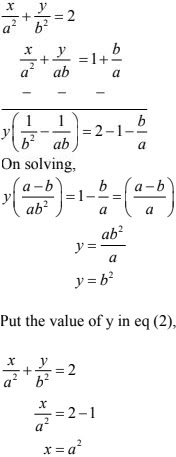10. Find the solution of the pair of equations x/10 + y/5 -1 = 0 and x/8 + y/6 =15. Hence, find λ, if y = λx + 5.
Solution
Given equations are
x/10 + y/5 -1 = 0 (Multiplying by 20)
⇒ 2x + 4y = 20  ...(i)
x/8 + y/6 =15 (Multiplying by 24)
⇒ 3x + 4y = 360 ...(ii)
On solving, equation (i) and (ii),
x = +340
Now,
x + 2y = 10
⇒ 340 + 2y = 10 [x = 340]
⇒ 2y = 10 – 340
⇒ 2y = –330
⇒ y = -165
Now,
y = λx + 5 [Given]
–165 = λ(340) + 5 [y = –165 and x = 340]
⇒ –λ(340) = 5 + 165
⇒ –λ(340) = 170
⇒ λ= -1/2
The solution of the given pair of equations is x = 340, y = –165 and λ= -1/2.

11. By the graphical method, find whether the following pair of equations are consistent or not. If consistent, solve them.
(i) 3x + y + 4 = 0 and 6x – 2y + 4 = 0
(ii) x – 2y = 6 and 3x – 6y = 0
(iii) x + y = 3 and 3x + 3y = 9
Solution
(i) Given equations are
3x + y + 4 = 0 ...(i)
6x – 2y + 4 = 0 ...(ii)
The given pair of equations is consistent and has unique solution.
3x + y + 4 = 0 [From (i)]
Y= -3x-4
If
x = 0,
⇒ y = –3(0) – 4 = 0 – 4 = –4
x = 1,
⇒ y = –3(1) – 4 = –3 – 4 = –7
x = 2
⇒ y = –3(2) – 4 = –6 – 4 = –10
Now,
6x – 2y + 4 = 0 [From (ii)]
⇒ 3x – y + 2 = 0
⇒ –y = –3x – 2
If x = 0,
⇒ y = 3(0) + 2 = 0 + 2 = 2
x = 1,
⇒ y = 3(1) + 2 = 3 + 2 = 5
x = 2,
⇒ y = 3(2) + 2 = 6 + 2 = 8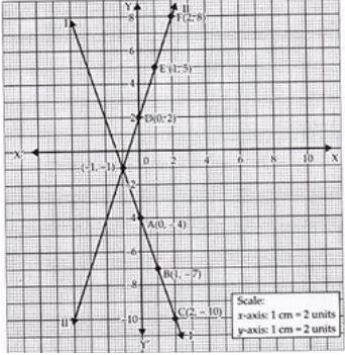Intersecting point is (–1, –1) i.e., x = –1 and y = –1

(ii) Given equations are,
x – 2y = 6 ...(i)
3x – 6y = 0 ...(ii)
System of equations is inconsistent. Hence, the lines represented by the given equations
are parallel. So, the given equations have no solution.

(iii) Pair of equations is
x + y = 3 ...(i)
3x + 3y = 9 ...(ii)
The system of given equations have infinitely many solutions. Graph will be overlapping
so pair of equations is consistent.
As the lines are dependent so points on graph for both equations will be same. To draw, we
can take any one equation.
x + y = 3
If x = 0,
⇒ y = 3 – 0 = 3
x = 1,
⇒ y = 3 – 1 = 2
x = 2,
⇒ y = 3 – 2 = 1So, the lines represented by the given equations are coinciding. Given equations are
consistent.
Some solutions of system of equations are consistent.
Some solutions of system of equations are (0, 3), (1, 2), (2, 1), (3, 0), (4, –1) and (5, –2).

12. Draw the graph of the pair of equations 2x + y = 4 and 2x – y = 4. Write the vertices of the triangle formed by these lines and the y-axis. Also find the area of this triangle.
Solution
2x + y = 4 ...(i)
y = 4 - 2x
If x = 0,
⇒ y = 4 – 2(0)
= 4 – 0 = 4
x = 1,
⇒ y = 4 – 2(1)
= 4 – 2 = 2
x = 2,
⇒ y = 4 – 2(2)
= 4 – 4 = 0
For,
x = 0, 1,2, 3
y = 4, 2,0 ,–2
Let the above four points be named as A, B, C, D
Now, 2x – y = 4 ...(ii)
If, x = 0,
⇒ y = 2(0) – 4
= 0 – 4 = –4
x = 1,
⇒ y = 2(1) – 4
= 2 – 4 = –2
x = 2,
⇒ y = 2(2) – 4
= 4 – 4 = 0
For,
x = 0,1 ,2, 3, 4
y = –4, –2, 0, 2, 4
Let the points be E, F, G, H ,I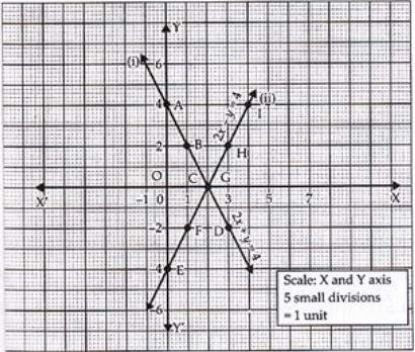Triangle formed by the lines with y–axis is ΔAEC. Coordinates of vertices are A(0, 4), E(0, –4)and C(2, 0).
Area of ΔAEC = 8 square units

13. Write an equation of a line passing through the point representing solution of the pair of linear equations x + y = 2 and 2x–y = 1. How many such lines can we find?
Solution
Given pair of linear equations is,
x + y = 2 ...(i)
2x – y = 1 ...(ii)
We have,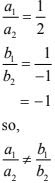So, the given pair of equations has unique solution.
For solution of equations, add (i) and (ii), we get
3x = 3
⇒ x = 1
Now, x + y = 2 [From (i)]
1 + y = 2
⇒ y = 1 [₹ x = 1]
So, the solution of the given equations is y = 1 and x = 1.
Now, we have to find a line passing through (1, 1). We can make infinite linear equations
passing through (1, 1). Some of the linear equations are given below:
Step I:
Take any linear polynomial in x and y, let it 8x – 5y.
Step II:
Put x = 1 and y = 1 in the above polynomial, i.e.
8(1) – 5(1) = 8 – 5 = 3
Step III:
The required equations are 2x–3y = -1, 3x–2y=1 and 5x–2y = 3 and x–y = 0 etc.

14. If x+1 is a factor of 2x2 + ax2  + 2bx + 1, then find the values of a and b given that 2a–3b = 4.
Solution
Let
f(x) = 2x3 + ax2 + 2bx + 1
If (x + 1) is a factor of f(x) , then by factor theorem f(-1) = 0.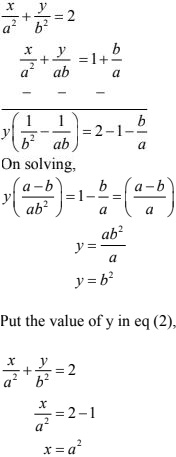b = 2

15. The angles of a triangle are x, y and 40°. The difference between the two angles x and y is 30°. Find x and y.
Solution
x, y and 40 are the measure of interior angles of a triangle.
x + y + 40° = 180°
⇒ x + y = 140° ...(i)
The difference between x and y is 30°,
x - y = 30°
x + y = 140°
⇒ 2x = 170°
⇒ x = 170°/2
⇒ x = 85°
Now,
x + y = 140° [From (i)]
⇒ 85° + y = 140° [x = 85°]
⇒ y = 140° - 85°
⇒ y = 55°
and x = 85°

16. Two years ago, Salim was thrice as old as his daughter and six years later, he will be four years older than twice her age. How old are they now?
Solution
Let the present ago of Salim be x years.
Also, let the present age of his daughter be y years.
Age of Salim 2 years ago = (x – 2) years
Age of Salim’s daughter 2 years ago = (y – 2) years
According to the question, we have
Age of Salim was = thrice × daughter [Given]
x – 2 = 3 × (y – 2)
⇒ x – 2 = 3y – 6
⇒ x – 3y = –4
Age of Salim 6 years later = (x + 6) years
Age of Salim’s daughter 6 years later = (y + 6) years
According to the question, we have
x + 6 = 2(y + 6) + 4
⇒ x + 6 = 2y + 12 + 4
⇒ x – 2y = 16 – 6
⇒ x - 2y =10
so,Now,
x - 2y = 10
⇒ x - 2(14) = 10 [₹ y = 14]
⇒ x = 10 + 28
⇒ x = 38
∴ Age of Salim at present = 38 years
and, age of Salim’s daughter at present = 14 years

17. The age of the father is twice the sum of the ages of his two children. After 20 years, his age will be equal to the sum of the ages of his children. Find the age of the father.
Solution
Let the present age of father be x years.
Also, let the sum of present ages of two children by y years.
The age of father is (=) twice (× 2) the sum of the ages of two children
x = 2 × (y)
⇒ x = 2y ...(i)
⇒ x – 2y = 0
Age of father 20 years later = (x + 20) years
Increase in age of first children in 20 years = 20 years
Increase in age of second children in 20 years = 20 years
∴ Increase in the age of both children in 20 years = 20 + 20 = 40 years
∴ Sum of ages of both children 20 years later = (y + 40)
Now, according to the question, we have
Father will be (=) sum of ages of two children [Given]
x + 20 = y + 40
⇒ x – y = 20 ...(ii)
⇒ 2y – y = 20 [( x = 2y) from (i)]
⇒ y = 20 years
Now,
x = 2y [From (i)]
⇒ x = 2 × 20
⇒ x = 40
So, Age of father is 40 years.

18. Two numbers are in the ratio 5 : 6. If 8 is subtracted from each of the numbers, the ratio becomes 4 : 5. Find the numbers.
Solution
Let the numbers be 5x and 6x respectively. So, new numbers after subtracting 8 fromeach
will be (5x – 8) and (6x – 8) respectively.
According to the question, ratio of new numbers is 4 : 5.
(5x - 8)/(6x - 5) = 4/5
⇒ 25x – 40 = 24x – 32
⇒ 25x – 24x = 40 – 32
⇒ x = 8
Required numbers = 5x and 6x become 5 × 8, 6 × 8
Required numbers = 40 and 48

19. There are some students in the two examination halls A and B. To make the number of students equal in each hall, 10 students are sent from A to B. But if 20 students are sent from B to A, the number of students in A becomes double the number of students in B. Find the number of students
in the two halls.
Solution
Let the number of students initially in hall A be x.
and the number of students initially in hall B be y.
Case I:
10 students of hall A shifted to B
Now, number of students in hall A = (x – 10)
Now, number of students in hall B = (y + 10)
According to the question, number of students in both halls are equal.
x – 10 = y + 10
⇒ x – y = 20 ...(i)
Case II:
20 students are shifted from hall B to A, then
Number of students in hall A becomes = x + 20
Number of students in hall B becomes = y – 20
According to the question, students in hall A becomes twice of students in hall B.
∴ x + 20 = 2(y – 20)
⇒ x + 20 = 2y – 40 ...(ii)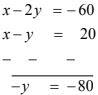y = 80
Now,
x - y = 20  [From (i)]
⇒ x – 80 = 20
⇒ x = 20 + 80
⇒ x = 100
Number of students initially in hall A = 100
Number of students initially in hall B = 80

20. A shopkeeper gives books on rent for reading. She takes a fixed charge for the first two days, and an additional charge for each day thereafter. Latika paid Rs 22 for a book kept for six days, while Anand paid Rs 16 for the book kept for four days. Find the fixed charges and the charge for each
extra day.
Solution
Let the fixed charges for first two days = ₹x
Let the additional charges per day after 2 days = ₹y
Latika paid ₹22 for six days. [Given]
2 days fixed charges + (6 – 2) days charges = 22
x + 4y = 22 ...(i)
Anand paid ₹16 for books kept for four days.
2 day’s fixed charges + (4 – 2) day’s additional charges = 16
x + 2y = 16 ...(ii)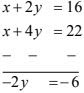y = ₹ 3 per day
Now,
x + 2y = 16 [From (ii)]
⇒ x + 2(3) = 16
⇒ x = 16 – 6
⇒ x = 10
So, the fixed charges for first 2 days = ₹10
The additional charges per day after 2 days = ₹3 per day

21. In a competitive examination, one mark is awarded for each correct answer while 1/2 mark is deducted for every wrong answer. Jayanti answered 120 questions and got 90 marks. How many questions did she answer correctly?
Solution
Taking the number of questions attempted correctly = x
Number of questions answered = 120
And, wrong answer attempted = (120 – x)
Marks awarded for right answer = 1 × x = x marks
Marks deducted for (120 – x) wrong answer = 1⁄2 (120 – x)
Therefore,
x - 1/2(120 - x) = 90
⇒ x + x/2 = 90 + 60
⇒ 3x/2 = 150
⇒ x = 100
Hence, Jayanti answered 100 questions correctly.

22. The angles of a cyclic quadrilateral ABCD are ∠A = (6x + 10) °, ∠B = (5x)° ∠C = (x + y)°, ∠D = (3y – 10)°
Solution
Sum of opposite angles of a cyclic quadrilateral ABCD is 180°,
∠A + ∠C = 180° [Opposite ∠s of cyclic quadrilateral)]
(6x + 10) + (x + y) = 180°
⇒ 6x + 10 + x + y = 180°
⇒ 7x + y = 170° ...(i)
Now,
∠B + ∠D = 180°
5x + (3y – 10) = 180°
⇒ 5x + 3y = 180° + 10°x = 20
Now,
7x + y = 170 [From (i)]
⇒ 7(20) + y = 170
⇒ y = 170 – 140
⇒ y = 30
and
x = 20
Therefore,
∠A = (6x + 10)°
= (6 × 20 + 10)°
= (120 + 10)° = 130°
∠B = (5x)°
= (5 × 20)°
= 100°
∠C = (x + y)°
= (20 + 30)°
= 50°
∠D = (3y – 10)°
= (3 × 30 – 10)°
= (90 – 10)°
= 80°
So, the values of x and y are 20 and 30 respectively.
∠A, ∠B, ∠C, and ∠D are 130°, 100°,50°, 80° respectively.